# What does ellipsoid

What is an ellipsoid?

 ...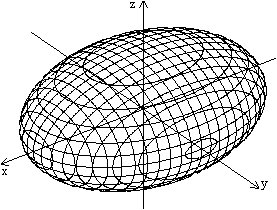An ellipsoid is the graph of the relation x² / a² + y² / b² + z² / c² = 1.The largest possible domain isD = {(x, y, z) | -a <= x <= a, -b <= y <= b, -c <= z <= c}It is shown here in the Cartesian coordinate system.For the drawing, x² / 4 + y² / 2 + z² / 1 = 1 applies.

 ...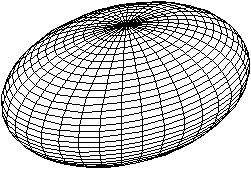The parametric representation has the same ellipsoidx = a * sin (u) cos (t)y = b * sin (u) sin (t)z = c * cos (u)D = {(t, u) | -2pi; <= t <= 2pi, -pi <= u <= pi}

It really is the same ellipsoid as the following calculation shows.
The following applies: x² / a² + y² / b² + z² / c²
= [a * sin (u) cos (t)] ² / a² + [b * sin (u) sin (t)] ² / b² + [c * cos (u)] ² / c² = sin² (u) cos² (t) + sin² (u) sin² (t) + cos² (u) = sin² (u) + cos² (u) = 1.

On my page Torus I explain how to draw bodies of this type with the program Winplot.

 ...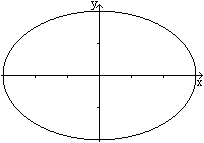The ellipsoid is the generalization of the ellipse to the third dimension.This is the graph of the relation x² / a² + y² / b² = 1 with D = {(x, y) | -a <= x <= a, -b <= y <= b}.

Designations Top
If you put a coordinate equal to zero in the coordinate equation x² / a² + y² / b² + z² / c² = 1, you get the three Main ellipses of the ellipsoid.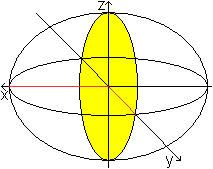x = 0 leads to the ellipse equation y² / b² + z² / c² = 1 with regard to the y-z plane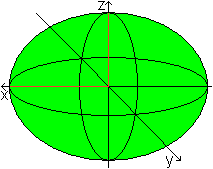y = 0 leads to the ellipse equation x² / a² + z² / c² = 1 with regard to the x-z plane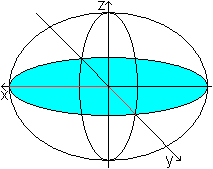z = 0 leads to the ellipse equation x² / a² + y² / b² = 1 with regard to the x-y plane

By the way, every plane section through the ellipsoid leads to an ellipse.

The ellipsoid is symmetrical to the main planes, since the term of the relation does not change if one replaces x, y and z with their opposite numbers -x, -y and -z.

 ...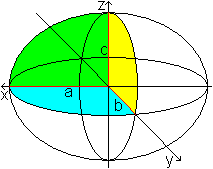The radiuses a, b and c of the main ellipses are also called Radius of the ellipsoid.If a> b> c, then a is the greatße, b the middle b and c the small radius. The vertices of the main ellipses are also called Parting of the ellipsoid.

An ellipsoid with a> b> c is called a three-axis ellipsoid.

Ellipsoids of revolution Top
Ellipsoids, where two out of three radiuses are the same, play a special role. For example, let a = b.
Then the equation (x² + y²) / a² + z² / c² = 1.
A distinction is made between two cases, namely c> a or c Elongated ellipsoid

 ...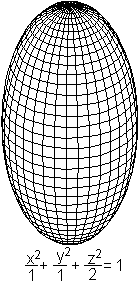... The body on the left has the equation (x² + y²) / a² + z² / c² = 1, where c> a is.If one sets z = 0, the equation becomes x² + y² = a².That is, a main ellipse becomes a circle and with it all parallel ellipses. The ellipsoid becomes rotationally or rotationally symmetrical.Rugby balls, for example, have this shape.
The body can also be interpreted as follows: A ball is stretched in the z-direction.

Flattened ellipsoid or spheroid
 ...... The body on the left has the equation (x² + y²) / a² + z² / c² = 1, where c is.If one sets z = 0, the equation becomes x² + y² = a².That means, a main ellipse becomes a circle and with it all parallel ellipses.The ellipsoid becomes rotationally or rotationally symmetrical.The earth is flattened like other celestial bodies in this way. This ellipsoid is also called a spheroid.Pastilles (e.g. Smarties) also have roughly this shape.
The body can also be interpreted as follows: A ball is compressed in the z-direction.

So one differentiates between the following ellipsoids.
 Triaxial ellipsoida Flattened ellipsoida = b, a Elongated ellipsoida = b, a> c Bulleta = b = c

Volume and surface Top
The volume and the surface of ellipsoids of revolution can be calculated elementarily.

If a curve rotates around the x-axis with y = f (x), the following two formulas apply for volume and surface area.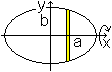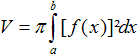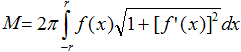Elongated ellipsoid
volume
 ...... So imagine that the ellipse with x² / a² + y² / b² = 1 or y² = (b² / a²) (a²-x²) rotates around the x-axis.Then applies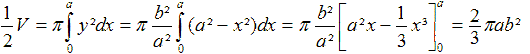surface
To calculate the second integral, one sets
> the equation x² / a² + y² / b² = 1,
> the derivative 2x / a² + 2 (y / b²) y '= 0 or y' = - (2b²x) / (2a²y) or y'² = (b4x2) / (a4y2) and
> the term sqrt (1 + y'²) = sqrt [1+ (b4x2) / (a4y2)] = ... = (b / y) sqrt [1- (ex / a²) ²] with e² = a²-b² ready.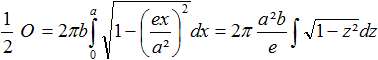The substitution is called z = ex / a², and it is dz = (e / a²) dx or dx = (a² / e) dz.

According to Bronstein (4, page 47) there is the following antiderivative for the integral.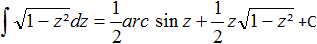Then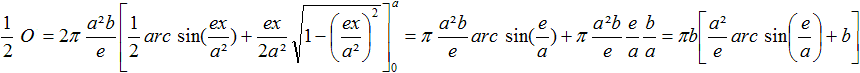Result
V = (4/3) pi * ab² and O = 2pi * b [b + a² / e * arc sin (e / a)] with e² = a²-b²

Flattened ellipsoid or spheroid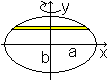V = (4/3) pi * a²b O = 2pi * a {a + b² / (2e) * ln [(a + e) ​​/ (a-e)]} with e² = a²-b²
Derivation of the two formulas: (1), pages 443 to 448 and pages 457 to 462

Triaxial ellipsoid
Ellipsoids are generally given by the semi-axes a, b and c. From this, the volume and the surface can be calculated. - I'll leave it at the formulas, for the surface I have to limit myself to an approximation.
 V = (4/3) pi * abc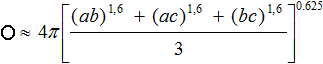found at de.wikipedia, more is available from Gérard P. Michon (URL below)

Second order surfaces Top
The ellipsoid belongs to the second order surfaces.
They result when the equation Ax + By + Cz + Dxy + Eyz + Fzx + Gx + Hy + Kz + L = 0 is graphically represented in a spatial Cartesian coordinate system.
With certain values ​​for the variables A to L, the following areas essentially result.

Ellipsoid on the internet Top

German

Lok Lam Mak (technical work by a 12th grade student)
The volume of a chicken egg

PiMath
THE SHAPE OF THE EARTH

Ralf Schaper (Department of Mathematics / Computer Science, University of Kassel)
Cut ellipsoid

Wikipedia
Ellipsoid, ellipsoid of revolution

English

F. W. PRESTON
The Volume of an Egg

Eric W. Weisstein (MathWorld)

Gerard P. Michon
Spheroids & Scalene Ellipsoids

Marlene Dieguez Fernandez

Richard Parris (freeware program WINPLOT)
The official website is closed. Download the German program e.g. from heise

The Wolfram Demonstrations Project
Ellipsoid, Eggnigmatica, EggnigmaticaII, Lamé's Ellipsoid And Mohr's Circles

Wikipedia
Ellipsoid, spheroid

Xahlee
Ellipsoid, rotate me

French

Robert FERRÉOL (mathcurve)
Ellipsoids

credentials Top
(1) Georg Ulrich / Paul Hoffmann: Differential and integral calculus for self-teaching, Hollfeld [ISBN 3 8044 0575 4]
Message from Torsten Sillke:
(2) Carlson, B.C., Special Functions of Applied Mathematics, pp. 261-272, Academic Press, New York (NY), 1977. N.B. In example 9.4-2, Carlson presents an elegant derivation of the surface area of ​​an ellipsoid in standard function form.

Feedback: Email address on my main page

URL of my homepage:
http://www.mathematische-basteleien.de/